# Fluid Mechanics - 4

## 20 Questions MCQ Test Mock Test Series for SSC JE Mechanical Engineering | Fluid Mechanics - 4

Description
Attempt Fluid Mechanics - 4 | 20 questions in 12 minutes | Mock test for SSC preparation | Free important questions MCQ to study Mock Test Series for SSC JE Mechanical Engineering for SSC Exam | Download free PDF with solutions
QUESTION: 1

### Run away speed of the hydraulic turbine is corresponding to speed if:

Solution:

The speed of hydraulic turbine if the runner of the turbine is allowed to run freely without any load and with the wicket gates wide opening is known as runaway speed. For a given type of turbine, Runaway speed differs due to design variations by different manufacturers. All the rotating parts in the turbine must be designed to withstand the stresses generated from the centrifugal forces at runaway speed.

QUESTION: 2

### The specific speed of a Francis turbine is in the range of:

Solution:

1. The specific speed of Pelton wheel turbine (single jet) is in the range of 10-35

2. The specific speed of Pelton wheel turbine (multiple jet) is in the range of 35-60

3. The specific speed of Francis turbine is in the range of 60-300.

4. The specific speed of Kaplan turbine is greater than 300.

QUESTION: 3

### The viscosity of liquid _________ with increase in temperature.

Solution:

For liquids the viscosity is given by:

μ=a10b/(T−C)μ=a10b/(T−C)

Where T is absolute temperature & a, b, c is experimentally determined constants.

For gasses: μ=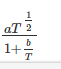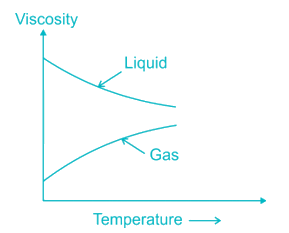QUESTION: 4

For Bernoulli’s equation to remain valid, which of the following is NOT required?

Solution:

The conditions to be satisfied for the applicability of Bernoulli’s equation are

I. Flow along a stream line

II. Flow is steady and Incompressible

III. Effect of viscous forces is negligible

QUESTION: 5

Which of the following causes the major loss in the long pipes?

Solution:

Head loss in a pipe flow problems are divided in to two parts:

Major head loss is due to friction

Some of the minor head losses are due to sudden enlargement, at the exit of pipe, due to sudden contraction, at the entrance of the pipe, due to pipe bend. But for long pipe flow problems major loss is due to head loss due to friction which is directly proportional to length of pipe.

QUESTION: 6

Which one of the following is true about ideal fluid?

Solution:

Ideal fluid is Incompressible and it has zero value of shear force. Ideal fluid does not actually exist in nature, but sometimes used for fluid flow problems. An ideal fluid is a fluid that has several properties including the fact that it is :

Incompressible – the density is constant

Irrotational – the flow is smooth, no turbulence

Nonviscous – (Inviscid) fluid has no internal friction

QUESTION: 7

Bluff body is the body of such a shape that pressure drag as compared to friction drag is

Solution: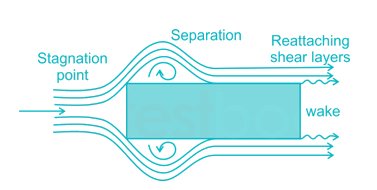A bluff body is defined as that body whose surface does not coincide with the streamlines when placed in a flow. Then the flow is separated from the surface of the body much ahead of its trailing edge with the result of a very large wake formation. Then the drag due to pressure will be very large as compared to the drag due to friction on the body.

QUESTION: 8

The most economical section of circular channel for maximum discharge is obtained when (Where, d is the diameter of circular section)

Solution:

D = depth of flow

d = diameter of pipe

Rm = Hydraulic Mean depth = A/P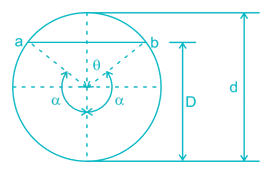Perimeter, P = αd

Condition for Maximum Discharge for Circular Section:

α = 154° = 2.65 radians

D = 0.95 d

Rm = 0.29 d

Condition for Maximum Velocity for Circular Section:

α = 128.75° = 2.25 radians

D = 0.81 d

Rm = 0.3 d

QUESTION: 9

The rise or depression of liquid in a tube due to surface tension with an increase in the size of the tube will

Solution:

Capillary Rise: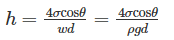So, the capillary rise or depression is inversely proportional to the diameter of the tube. So when the size is increased, the capillary rise will decrease

QUESTION: 10

ν=0.0022t−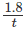is the equation to determine kinematic viscosity of liquids by

Solution:

The device used for measurement of viscosity is known as viscometer. According to Saybolt viscometer:

ν=μρ=0.0022t−QUESTION: 11

Fluid is a substance which offers no resistance to change of

Solution:

A fluid can be defined as a substance which is capable of flowing and changing its shape according to the surrounding without offering internal resistance. Liquid offers no resistance to change of shape and it conforms to the shape of its surrounding.

QUESTION: 12

The volumetric change of the fluid caused by a resistance is ________

Solution:

In fluids, Compressibility is a measure of the relative volume change of a fluid as a response to as pressure change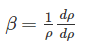Where β Compressibility

ρ density of a fluid

p ⇒ pressure acting

QUESTION: 13

The boundary layer is formed as the flowing fluid comes in contact with the solid surface, because of the action of:

Solution:

A boundary layer is a thin layer of viscous fluid close to the solid surface of a wall in contact with a moving stream in which (within its thickness δ) the flow velocity varies from zero at the wall (where the flow “sticks” to the wall because of its viscosity) up to U (free stream velocity) at the boundary. In a flow field, viscous stresses are very prominent within this layer.

QUESTION: 14

An impulse turbine produces 50 kW of power when the blade mean speed is 400 m/s. What is the rate of change of momentum tangential to the rotor?

Solution:

Power=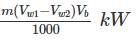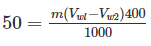m (Vw1 - Vw2) = rate of change of momentum tangential to the rotor ​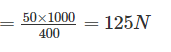QUESTION: 15

The cipoletti weir functions as if it were a following notch without end contractions

Solution:

The "Cippoletti" weir is a trapezoidal weir, having 1 horizontal to 4 vertical side slopes, as shown in figure. The purpose of the slope, on the sides, is to obtain an increased discharge through the triangular portions of the weir, which, otherwise would have been decreased due to end contractions in the case of rectangular weirs.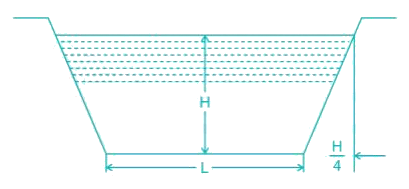QUESTION: 16

Friction drag is generally larger than the pressure drag in _______.

Solution:

A body moving through a fluid experiences a drag force, which is usually divided into two components: frictional drag, and pressure drag.

Frictional drag comes from friction between the fluid and the surfaces over which it is flowing. This friction is associated with the development of boundary layers, and it scales with Reynolds number.

Pressure drag comes from the eddying motions that are set up in the fluid by the passage of the body. This drag is associated with the formation of a wake, which can be readily seen behind a passing boat, and it is usually less sensitive to Reynolds number than the frictional drag. It depends on the shape of the body.

Frictional drag is important for attached flows (that is, there is no separation), and it is related to the surface area exposed to the flow. Pressure drag is important for separated flows, and it is related to the cross-sectional area of the body.

For streamlined bodies (like a fish, or an airfoil at small angles of attack), frictional drag is the dominant source of air resistance. For a bluff body (like a brick, a cylinder, or an airfoil at large angles of attack), the dominant source of drag is pressure drag.

QUESTION: 17

A hydraulic intensifier normally consists of _____.

Solution:

A hydraulic intensifier is a device which is used to increase the intensity of pressure of any hydraulic fluid or water, with the help of the hydraulic energy available from a huge quantity of water or hydraulic fluid at a low pressure. These devices are very important in the case of hydraulic machines, mainly hydraulic presses, which require water or hydraulic fluid at a very high pressure which cannot be obtained from the main supply directly. There are three main parts in the hydraulic intensifiers to be noted. They are

• Fixed ram
• Hollow inverted sliding cylinder
• Fixed inverted cylinder
•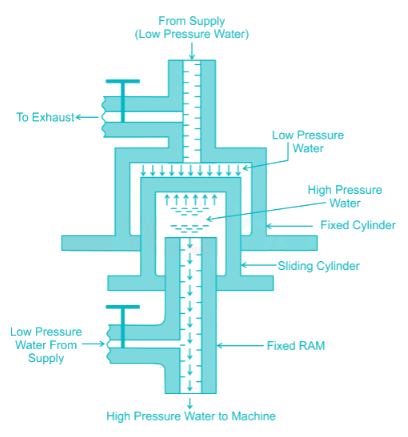QUESTION: 18

Critical-depth meter is used to measure _____.

Solution:

For a given value of specific energy, the critical depth gives the greatest discharge in an open channel, or conversely, for a given discharge, the specific energy is a minimum for the critical depth. So at a control section, the discharge can be calculated once the depth is known.

The critical depth is given as,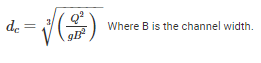QUESTION: 19

The force exerted by the jet on fixed plate shown in the figure below is equal to: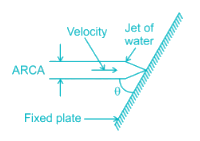Solution: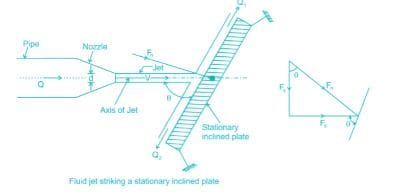Let us apply the impulse-momentum equation in the direction normal to the plate

Fn=ρaV(Vsinθ−0)=ρaV2sinθFx=Fnsinθ=ρV2sinθ×sinθ=ρaV2sin2θFy=Fncosθ=ρV2sinθ×cosθ=ρaV2sin2θcosθ

QUESTION: 20

The energy loss in flow through nozzle as compared to venturimeter is

Solution:

The flow nozzle is essentially a venturimeter with the divergent part omitted. Therefore the basic equations for calculation of flow rate are the same as those for a venturimeter. The dissipation of energy downstream of the throat due to flow separation is greater than that for a venturimeter. But this disadvantage is often offset by the lower cost of the nozzle. Flow nozzle(top) and venturimeter(bottom) diagram is given below.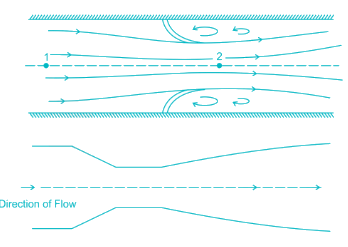Use Code STAYHOME200 and get INR 200 additional OFF Use Coupon Code